## Data manipulations with {dplyr}

Function name Action
select()
returns a subset of the columns (variables)
mutate()
used to add columns (variables) from existing data
filter()
returns a subset of the rows (observations)
arrange()
reorders the rows according to single or multiple variables
summarize()
reduces each group to a single row by calculating aggregate measures

## Helper functions, inside a select()

Function name Description
starts_with("X")
every name that starts with "X"
ends_with("X")
every name that ends with "X"
contains("X")
every name that contains "X"
matches("X")
every name that matches "X", where "X" can be a regular expression
num_range("x", 1:5)
the variables named x01, x02, x03, x04 and x05
one_of(x)
every name that appears in x, which should be a character vector

## Helper functions, inside a filter()

x < y,   …  ,  x != y,    is.na()

x  %in%  c(a, b, c)

``````filter(df, !is.na(x) | Distance>=3000)
filter(df, Aeroport %in% c("Domodedovo", "BenGurion", "Delta"))
filter(df, DayOfWeek %in% c(6,7), Distance > 1000)
``````

## arrange(), mutate()

``````arrange(hflights, UniqueCarrier, desc(DepDelay))
arrange(hflights, DepDelay+ArrDelay)
mutate(df, Date=paste(Year, Month, DayofMonth, sep='-'))``````
``` ```
``` ```
``` summarize() Aggregate functions to use within: min, max, sum, mean, sd, var, median, length first, last, nth(x,n) n() – the number of rows in the data.frame or group of observations that summarize() describes n_distinct(x) – The number of unique values in vector x quantile(x, p) – pth quantile of vector x diff(range(x)) – total range of vector x IQR() – Inter Quartile Range (IQR) of vector x summarize(df, min_dist=min(Distance), max_dist=max(Distance)) summarize(filter(df, Diverted>0), max_div=max(Distance)) # Generate summarizing statistics for hflights summarize(hflights, n_obs = n(), n_carrier = n_distinct(select(hflights, UniqueCarrier)), n_dest = n_distinct(select(hflights, Dest))) # All American Airline flights aa <- filter(hflights, UniqueCarrier == "American") # Generate summarizing statistics for aa summarize(aa, n_flights= n(), n_canc=sum(Cancelled==1), avg_delay=mean(ArrDelay, na.rm=TRUE)) Pipes %>% #The following two are equivalent: mean(c(1, 2, 3, NA), na.rm = TRUE) c(1, 2, 3, NA) %>% mean(na.rm = TRUE) group_by(), rank() rank() takes a group of values and calculates the rank of each value within the group ```
``` ```
``` ```
``` ```
``` ```
``` ```
``` Remarks tbl is a special kind of data.frame. It makes data easier to look at and to work with; does not change the data’s structure. tbl1 <- as_tibble(df) tbl(my_db, "dplyr") *** When you refer to columns directly inside select(), filter(), you don’t use quotes.If you use the helper functions, you do use quotes. Joining Data Mutating joins {dplyr} join functions: — Always preserve row order — Can be applied to databases, spark, etc. left_join(), right_join() bands2 <- left_join(bands, artists, by = c("first", "last")) bands3 <- right_join(artists, bands, by = c("first", "last")) setequal(bands2, bands3)#TRUE inner_join() only retains observations that appear in both datasets inner_join(songs, albums, by = "album") full_join() returns all of the data that appears in both datasets Often resulting in many missing values artists %>% full_join(bands, by = c("first", "last")) artists %>% Filtering joins Semi-joins provide a useful way to explore the connections between multiple tables of data. semi_join(songs, by = c("first", "last")) # Collect the albums made by a band # Count the albums made by a band albums %>% semi_join (bands, by = "band") %>% nrow() anti_join() The way to check for missplellings in the key values, to diagnose joins that go wrong. Anti-joins provide a useful way to reason about how a mutating join will work before you apply the join. For example, you can use an anti-join to see which rows will not be matched to a second dataset by a join.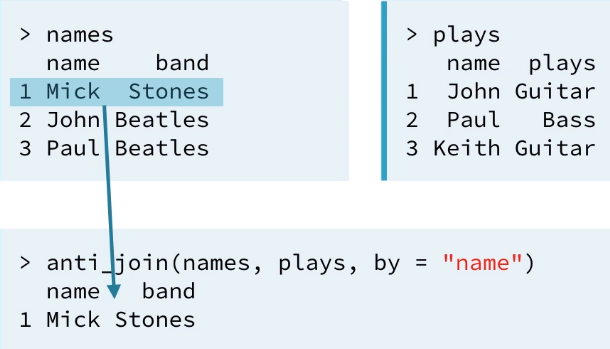Set operations union() function provides an easy way to combine two datasets without duplicating any values. intersect() is the set operator equivalent of a semi-join setdiff() Comparisons identical() determines whether dataframes contain the same items in the same order. setequal() determines whether dataframes contain the same items in any order. Remarks *When your datasets contain the same variables, a setdiff() does the same thing as an anti_join() that uses every column as a key. bind_cols(), bind_rows() vs cbind(), rbind()bind_cols(), bind_rows() are faster, can take a list of data frames as input, always return a tibble (a df with class tibble_df ). rbind() returns an error when column names don’t match across data frames. bind_rows() creates a column for each unique column name and distributes missing values as appropriate.Remarks If bind_cols() binds two datasets whose rows do not align, the meaningless data will be created. Unfortunately, there is usually no clear way to tell whether or not the rows in two datasets align unless the datasets contain a mutual key. In that scenario, you can use a mutating join to bind the datasets in a foolproof way.When you have multiple datasets in a list, you can use the bind_rows() function to bind all datasets into a single data frame. data_frame(), as_data_frame() data.frame() data_frame() {dplyr} Changes string to factors Doesn't change row/column names, doesn't change the data type of vectors Adds row names Input is a set of named vectors, separated by commas Changes unusual column names Output is tbl_df class, a data frame that contains vectors as columns. You can refer a one column to build a following column: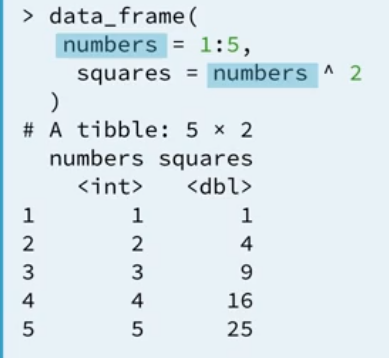Data types and coercion rules R coercion rules: R converts the more specific type of data into the more general: Combining any types of data with a character string will be coerced to a character string Combining integer and logicals with double will be coerced to a double Logical + Integer = Integer as.character() will coerce factors to their labels as strings if strings are present. as.numeric() will coerce factors to their levels as numerics if numerics are present. Each column of a data frame is stored as a single vector ==> must be a single type or class of data. OMG Factors Coercing factors can create a surprise because factors are often saved with numeric values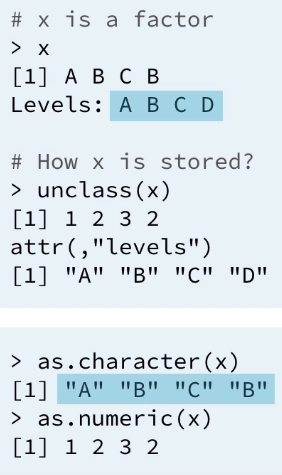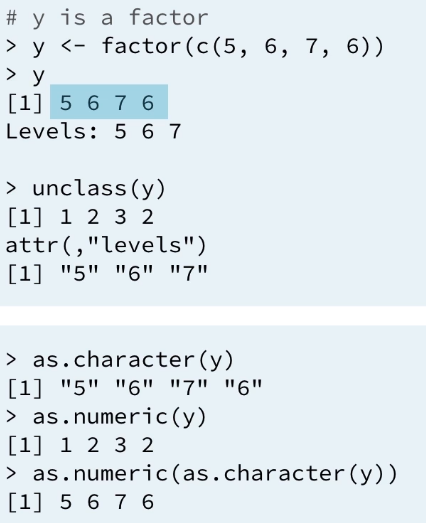{dplyr}'s coercion behavior dplyr functions will not automatically coerce data types Returns an error Expects you to manually coerce data Exceprion: factors dplyr converts non-aligning factors to strings (and then it will combine the data)Gives warning message Never apply as.numeric() to factors. First coerce to character:seventies %>% # Coerce seventies\$year into a useful numeric mutate(year = as.numeric(as.character(year))) What can go wrong? Missing / duplicate key values / key columns. Missing key value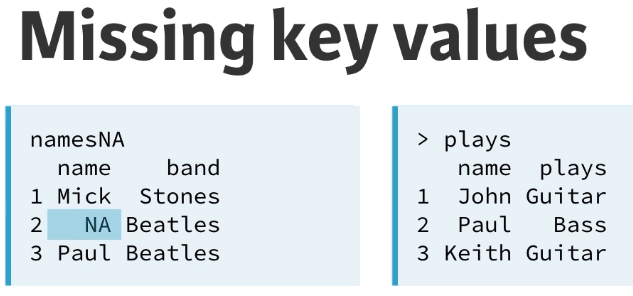There is no anything you can do to match this row to the secondary dataset namesNA %>% filter(!is.na(name)) %>% left_join(plays, by="name") Missing key column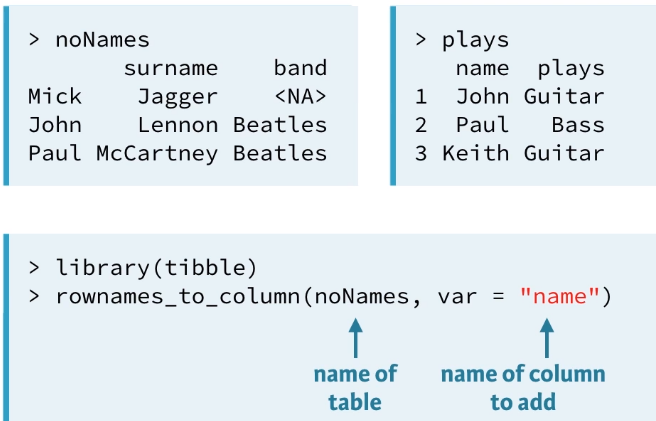Duplicate key value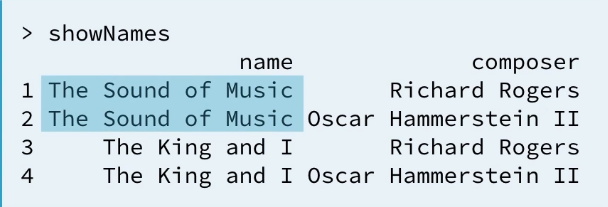Dplyr’s join functions will still work in this case. Dplyr will return every combinations of key value mathes across datasets. Mismatched key names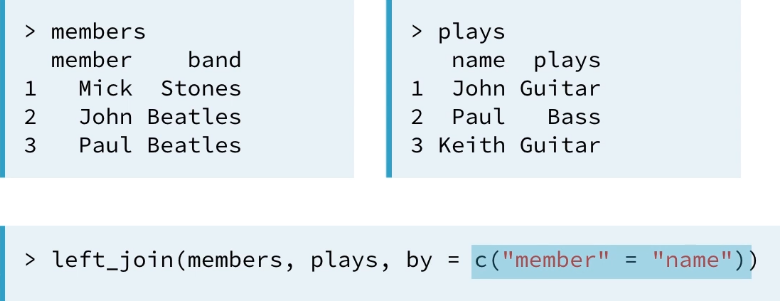Conflicting names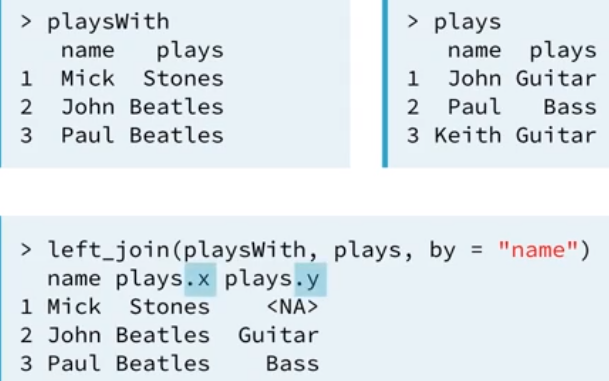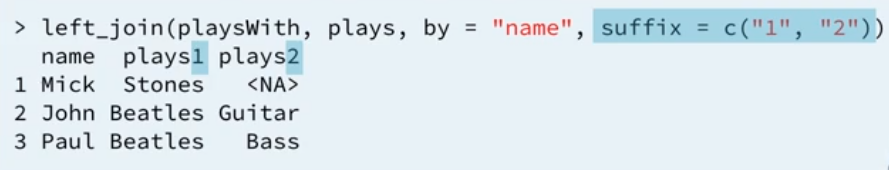Another way to setting a suffix or to completely rename the column names: left_join(x, y) %>% rename("plays1"="plays.x", "plays2"="plays.y") To make the join by two columns with mismatched key names (differents column names for key column) x %>% left_join(y, by = c("x.name1" = "y.name2")) Joining multiple tables reduce() {purrr}Remarks {purrr} rounds out the functional programming tools in R, helps to apply R functions to data in efficient ways. ```
``` ```
``` ```
``` ```
``` ```
``` Recent Posts WordPress Resources at SiteGround Hello world! Archives June 2018 June 2020 M T W T F S S « Jun     1234567 891011121314 15161718192021 22232425262728 2930   ©2020 * WebMust /* <![CDATA[ */ var localize = {"ajaxurl":"https:\/\/webmust.org\/wp-admin\/admin-ajax.php"}; /* ]]> */ var ElementorProFrontendConfig = {"ajaxurl":"https:\/\/webmust.org\/wp-admin\/admin-ajax.php","nonce":"bd472587bb","shareButtonsNetworks":{"facebook":{"title":"Facebook","has_counter":true},"twitter":{"title":"Twitter"},"google":{"title":"Google+","has_counter":true},"linkedin":{"title":"LinkedIn","has_counter":true},"pinterest":{"title":"Pinterest","has_counter":true},"reddit":{"title":"Reddit","has_counter":true},"vk":{"title":"VK","has_counter":true},"odnoklassniki":{"title":"OK","has_counter":true},"tumblr":{"title":"Tumblr"},"delicious":{"title":"Delicious"},"digg":{"title":"Digg"},"skype":{"title":"Skype"},"stumbleupon":{"title":"StumbleUpon","has_counter":true},"telegram":{"title":"Telegram"},"pocket":{"title":"Pocket","has_counter":true},"xing":{"title":"XING","has_counter":true},"whatsapp":{"title":"WhatsApp"},"email":{"title":"Email"},"print":{"title":"Print"}},"facebook_sdk":{"lang":"en_US","app_id":""}}; var elementorFrontendConfig = {"environmentMode":{"edit":false,"wpPreview":false},"is_rtl":false,"breakpoints":{"xs":0,"sm":480,"md":768,"lg":1025,"xl":1440,"xxl":1600},"version":"2.5.16","urls":{"assets":"https:\/\/webmust.org\/wp-content\/plugins\/elementor\/assets\/"},"settings":{"page":[],"general":{"elementor_global_image_lightbox":"yes","elementor_enable_lightbox_in_editor":"yes"}},"post":{"id":589,"title":"dplyr","excerpt":""}}; ```